Courses

# Test: Heat Capacity

## 17 Questions MCQ Test Chemistry for JEE | Test: Heat Capacity

Description
This mock test of Test: Heat Capacity for JEE helps you for every JEE entrance exam. This contains 17 Multiple Choice Questions for JEE Test: Heat Capacity (mcq) to study with solutions a complete question bank. The solved questions answers in this Test: Heat Capacity quiz give you a good mix of easy questions and tough questions. JEE students definitely take this Test: Heat Capacity exercise for a better result in the exam. You can find other Test: Heat Capacity extra questions, long questions & short questions for JEE on EduRev as well by searching above.
QUESTION: 1

Solution:
QUESTION: 2

### A rather soft, silvery metal was observed to have a specific heat of 0.225 cal K-1g-1. Thus, metal is

Solution:

It should be better to remove this question. It's data based.

QUESTION: 3

### Specific heat of water is 4.184 JK-1g-1, Rise in temperature when 1 kJ of heat is absorbed by 2 moles of H2O is

Solution:

We have,
Q = ms∆T
1000 = 2*18*4.184*∆T
∆T = 6.63 K

QUESTION: 4

The amount of the heat released when 20 ml 0.5 M NaOH is mixed with 100 ml 0.1 M HCl is x kJ. The heat of neutralization is

Solution: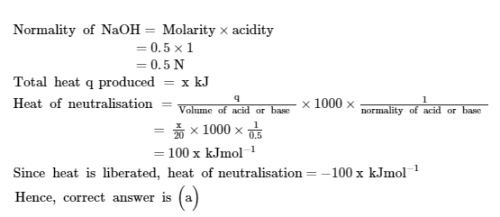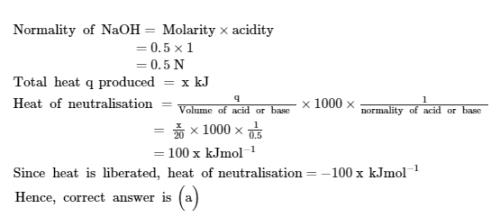QUESTION: 5

The heat capacity ratio, r was determined for cyanogen as 1.177. Thus, Cp for this gas is

Solution:

γ = 1.177
CV = R/γ-1
= 8.314/0.177 = 46.97
Also γ = CP/CV
1.177 = CP/46.97
​Or CP = 55.28

QUESTION: 6

Assuming the composition of air to be X (N2) = 0.80, X (O2) = 0.18 and X (CO2) = 0.02, molar heat capacity of air at constant pressure is

Solution:

(Cp)mixture = (µ1Cp12Cp23Cp3+.........)/µ123+.........   where µ is the no of moles.
Or (Cp)mixture = ƞ1Cp12Cp23Cp3+.............  Where ƞ is the corr. mole fraction.
On putting the values, we have
Cpmixture= ( 7/2*0.8 +7/2*0.18 +4*0.02)R
=3.15R

QUESTION: 7

Latent heat of fusion of ice is 6.02 kJ mol-1. The heat capacity of water is 4.18 Jg-1K-1. 500 g of liquid water is to be cooled from 20°C to 0°C . Number of ice cubes (each of one mole) required is

Solution:

Molecular mass of hydrogen = 1

Molecular mass of oxygen =16

Molecular mass of water = 18 gram per mole

As,

n = m / M

n = 500 / 18

n = 27.8 moles

For cooling 27.8 moles at 20 = 27.8 x 75.4 x 20 = 41.922 kilo joule

41.922/6  = 6.987 that is approximately equal to 7

So, the number of ice cubes that are required to cool the water will be 7.

QUESTION: 8

Sulphur (2.56 g) is burned in a constant volume calorimeter with excess O2(g). The temperature increases from 21.25°C to 26.72°C . The bomb has a heat capacity of 923 JK-1. Calorimeter contains 815 g of water. Thus, change in internal energy per mole of SO2 formed for the reaction is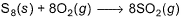(specific heat of water is 4.184 JK-1g-1.)

Solution:

Moles of S8 = 2.56/256 = 0.01
So, moles of SO2  formed = 0.08
Rise in temperature = (273+26.72)K - (273+21.25)K
= 5.47 K
Total Energy in internal energy (as system is at constant volume) = -(923 jK-1 × 5.47 +815g×4.18)
= -23701.29 J = -23.70129 kJ
Thus change in internal energy per mole of formed = -23.7012kJ/0.08 = -296.3 kJ

QUESTION: 9

Direction (Q. Nos. 9) This sectionis based on statement I and Statement II. Select the correct answer from the code given below.

Q.

Statement I : Cv value of helium (He) is always 3/2R but Cv value of hydrogen (H2) is 3/2R at low temperature, 5/2R at moderate temperature and more than 5/2R at higher temperature.

Statement II : At lower temperature, only translational degree of freedom contributes to heat capacity while at higher temperature, rotational and vibrational degrees of freedom also contribute to heat capacity.

Solution:

In case of helium (monoatomic gas) we have only three degrees of freedom which correspond to three translational motion so the total heat capacity will increase. The contributors by vibrational motion are not appreciable at low temperature but increase from 0 to R when temperature increases.
CV=−f(R)/2
CV=-f(R)2, where f is the degree of freedom. At low temperature only translational motion is considered and f=3
∴CV=3R/2
f=3.∴CV=3R2 At moderate temperature both translational and rotational motions are considered.
f=3+2
f=3+2 (3-translational and 2 rotational).
∴CV=5R/2
∴CV=5R2. At still high temperature translational, rotational and vibrational motions are considered.
f=3+2+2
f=3+2+2 (3-translatinal , 2-rotational, 2-vibrational).
∴CV=7R/2

*Multiple options can be correct
QUESTION: 10

Direction (Q. Nos. 10-11) This section contains 2 multiple choice questions. Each question has four
choices (a), (b), (c) and (d), out of which ONE or  MORE THANT ONE  is correct.

Q. Select the correct alternate(s).

Solution:
*Multiple options can be correct
QUESTION: 11

Select the correct alternate(s).

Solution:

CP-CV = R = 2 cal
CP-CV = [3+(2x10-2)T cal mol-1 K-1] -  [1+(2 x 10-2)T cal mol-1 K-1] = 2
∆H = CP(T2-T1) = (CV + R)(T2-T1)
∆E = CV(T2-T1) = (CP-R)(T2-T1)

*Answer can only contain numeric values
QUESTION: 12

Direction (Q. Nos. 12 - 14) This section contains 3 questions. when worked out will result in an integer from 0 to 9 (both inclusive).

Q. An 88.5 g piece of iron whose temperature is 352.0 K is placed in a beaker containing 244 g of water at 292.0 K. When thermal equilibrium is reached, what is the change in the temperature of water?
Specific heat of water and iron are (4.184 Jg-1 K-1) and (0.449 Jg-1 K-1), respectively.

Solution:

Heat lost by iron = Heat gained by water
Let the equilibrium temperature be T.
mIronsIron (352-T) = mwaterswater(T-292)
88.5×0.449×(352-T) = 244×4.18×(T-292)
On calculation approximation,
40(352-T) = 1020(T-292)
0.04(352-T) = (T-292)
T = 306/1.04 = 294 K
So, change in temperature of water = 294-292 = 2K

*Answer can only contain numeric values
QUESTION: 13

The heat capacity of a calorimeter (commonly called the calorimeter constant) was determined by heating the calorimeter and its content using an electrical heater. If ΔT = 1.221 K as 1.25 A of electricity at 3.26 V was passed through the heater immersed in 137.5 g of water in the calorimeter for 175 s, determine the calorimeter constant (in JK-1). (Specific heat of water at constant pressure is 75.291 JK-1 mol-1).

Solution:

Subtracting the energy gained by the cold water from the energy lost by the hot water. This will give us the amount of energy gained by the calorimeter. Dividing the energy gained by the calorimeter by Tc (the temperature change of the cold water). This final answer is calorimeter constant
ms(dT)=137.5×4.184×1.221=702
joules=coulomb×volt =. 1.25×175×3.26=713
713 - 702 = 11
(11/1.221) = 9

*Answer can only contain numeric values
QUESTION: 14

In a constant volume calorimeter, 3.5 g of a gas (molar mass = 28 g mol-1) was burnt in excess oxygen at 298.0 K. The temperature of the calorimeter was found to increase from 298.0 K to 298.45 K due to oxidation of the gas. Given that the heat capacity of the calorimeter is 2.5 kJ K-1, the numerical value of the heat given out in kJ mol-1 is

[IIT JEE 2009]

Solution:

Energy released by combustion of 3.5 g gas = 2.5×(298.45-298) kJ
Energy released by 1 mole of gas = 2.5×0.45/3.5/28 = 9 kJ mol-1

QUESTION: 15

Direction (Q. Nos. 15-16) This section contains a paragraph, wach describing theory, experiments, data etc. three Questions related to paragraph have been given.Each question have only one correct answer among the four given options (a),(b),(c),(d).

Boiling point of ethanol (C2H5OH) = 78.29°C
Specific heat of ethanol = 2.44 J-1K-1
Enthalpy of vaporisation = 855 Jg-1

Q. Energy required (in kJ) for the following change is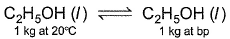Solution:

Q = ms∆T
= 1000(2.44×58.29)
= 997.23 kJ
As we have been asked till boiling point. But the compound is still in a liquid state. So mL is not given

QUESTION: 16

Boiling point of ethanol (C2H5OH) = 78.29°C
Specific heat of ethanol = 2.44 J-1K-1
Enthalpy of vaporisation = 855 Jg-1

Q. Energy required (in kJ) for the following change is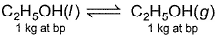Solution:

As liquid is already at boiling point initially and after giving heat it still is at boiling point. It means that only latent heat is given.
Q = mL = 1000×855 = 855 kJ

QUESTION: 17

Direction (Q. Nos. 17) Choice the correct combination of elements and column I and coloumn II  are given as option (a), (b), (c) and (d), out of which ONE option is correct.

Q. According to the classical equipartition of energy theory, the contributions to the molar heat capacity at constant volume are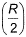for each degree of translational freedom,for each degree of rotational freedom and (R) for each degree of translational freedom. Match the species in Column I with the values of molar heat capacity (Cp or CV) in Column II and select answer from codes given below the table.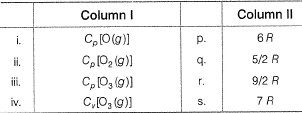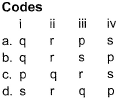Solution:

For monoatomic gas, CP = 5R/2
For diatomic gas, CP = 9R/2
For triatomic gas, CV = 6R and CP = CV+R = 7R